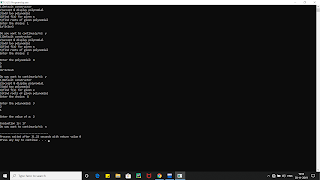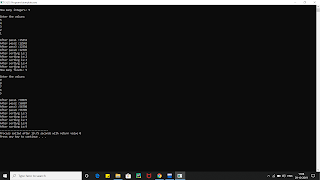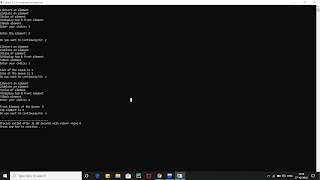## Posts

Showing posts from 2019

### Implement a class Quadratic that represents degree two polynomials i.e., polynomials of type ax2+bx+c. The class will require three data members corresponding to a, b and c. Implement the following operations: 1. A constructor (including a default constructor which creates the 0 polynomial). 2. Overloaded operator+ to add two polynomials of degree 2. 3. Overloaded << and >> to print and read polynomials. To do this, you will need to decide what you want your input and output format to look like. 4. A function eval that computes the value of a polynomial for a given value of x. 5. A function that computes the two solutions of the equation ax2+bx+c=0.Implement a class Quadratic that represents degree two polynomials i.e., polynomials of type ax2+bx+c. The class will require three data members corresponding to a, b and c. Implement the following operations: 1. A constructor (including a default constructor which creates the 0 polynomial). 2. Overloaded operator+ to add two polynomials of degree 2. 3. Overloaded << and >> to print and read polynomials. To do this, you will need to decide what you want your input and output format to look like. 4. A function eval that computes the value of a polynomial for a given value of x. 5. A function that computes the two solutions of the equation ax2+bx+c=0. OUTPUT FOR THE PROGRAM #include<iostream> #include<cmath> using namespace std; class polynomial { int a,b,c; public: polynomial() { a=b=c=0; } polynomial(int x,int y,int z) { a=x; b=y; c=z; } polynomial operator +(polynomial T) { polynomial R; R.a=a+T.a; R.

### Write a function template selection Sort. Write a program that inputs, sorts and outputs an integer array and a float array.Write a function template selection Sort. Write a program that inputs, sorts and outputs an integer array and a float array. #include<iostream> OUTPUT FOR THE PROGRAM using namespace std; #define size 10 int n; template<class T> void selection(T a[size]) { int i,j,k; T temp; for(i=0;i<n-1;i++) { for(j=i+1;j<n;j++) { if(a[j]<a[i]) { temp=a[i]; a[i]=a[j]; a[j]=temp; } } cout<<"\nAfter pass"<<i+1<<" :"; for(k=0;k<n;k++) { cout<<a[k]; } } for(i=0;i<n;i++) { cout<<"\nAfter sorting is:"<<a[i]; } } int main() { int i; int a[size]; float b[size]; cout<<"\nHow many integers: "; cin>>n; cout<<"\nEnter the values: \n"; for(i=0;i<n;i++) { cin>>a[i]; } selection(a); cout<<"\nHow many floats: "; cin>>n; cout<<&q

### Create User defined exception to check the following conditions and throw the exception if the criterion does not meet. a. User has age between 18 and 55 b. User stays has income between Rs. 50,000 – Rs. 1,00,000 per month c. User stays in Pune/ Mumbai/ Bangalore / Chennai d. User has 4-wheeler Accept age, Income, City, Vehicle from the user and check for the conditions mentioned above. If any of the condition not met then throw the exception.Create User defined exception to check the following conditions and throw the exception if the  criterion does not meet. a. User has age between 18 and 55 b. User stays has income between Rs. 50,000 – Rs. 1,00,000 per month c. User stays in Pune/ Mumbai/ Bangalore / Chennai d. User has 4-wheeler Accept age, Income, City, Vehicle from the user and check for the conditions mentioned above. If any of the condition not met then throw the exception. OUTPUT FOR THE PROGRAM #include<iostream> #include<string.h> using namespace std; int main() { int age; double income; char city; long int vehicle; cout<<"\nEnter the age: "; cin>>age; cout<<"\nEnter the income: "; cin>>income; cout<<"\nEnter the city: "; cin>>city; cout<<"\nEnter the vehicle(2/4): "; cin>>vehicle; try { if(age<18 || age>55) throw age; else cout<<"

### A book shop maintains the inventory of books that are being sold at the shop. The list includes details such as author, title, price, publisher and stock position. Whenever a customer wants a book, the sales person inputs the title and author and the system searches the list and displays whether it is available or not. If it is not, an appropriate message is displayed. If it is, then the system displays the book details and requests for the number of copies required. If the requested copies book details and requests for the number of copies required. If the requested copies are available, the total cost of the requested copies is displayed; otherwise the message ?Required copies not in stock? is displayed. Design a system using a class called books with suitable member functions and Constructors. Use new operator in constructors to allocate memory space required. Implement C++ program for the systemA book shop maintains the inventory of books that are being sold at the shop. The list includes  details such as author, title, price, publisher and stock position. Whenever a customer wants a  book, the sales person inputs the title and author and the system searches the list and displays  whether it is available or not. If it is not, an appropriate message is displayed. If it is, then the  system displays the book details and requests for the number of copies required. If the  requested copies book details and requests for the number of copies required. If the requested  copies are available, the total cost of the requested copies is displayed; otherwise the message ?Required copies not in stock? is displayed. Design a system using a class called books with  suitable member functions and Constructors. Use new operator in constructors to allocate  memory space required. Implement C++ program for the system. OUTPUT FOR THE PROGRAM #include<iostream> #include<string.h&g

### Write a C++ program create a calculator for an arithmetic operator (+, -, *, /). The program should take two operands from user and performs the operation on those two operands depending upon the operator entered by user. Use a switch statement to select the operation. Finally, display the result. Some sample interaction with the program might look like this: Enter first number, operator, second number: 10 / 3 Answer = 3.333333 Do another (y/n)? y Enter first number, operator, second number: 12 + 100 Answer = 112 Do another (y/n)? nWrite a C++ program create a calculator for an arithmetic operator (+, -, *, /). The program should take two operands from user and performs the operation on those two operands depending upon the operator entered by user. Use a switch statement to select the operation. Finally, display the result. Some sample interaction with the program might look like this: Enter first number, operator, second number: 10 / 3 Answer = 3.333333 Do another (y/n)? y Enter first number, operator, second number: 12 + 100 Answer = 112 Do another (y/n)? n OUTPUT FOR THE PROGRAM #include<iostream> using namespace std; int main() { int i,j,ans; float div; char ch,con; cout<<"\nEnter first number: "; cin>>i; cout<<"\nEnter second number: "; cin>>j; do { cout<<"\nEnter the operator(+,-,/,*): "; cin>>ch; switch(ch) { case '+': { ans=i+j; cout<<"\nAddition

### Write a c++ program to implement deque using STLWrite a c++ program to implement deque using STL. OUTPUT FOR THE PROGRAM #include <iostream> #include <deque> using namespace std; int main() {     deque<int> dq;     deque<int>::iterator it;     int choice, item;     while (1)     {         cout<<"\n---------------------"<<endl;         cout<<"Deque Implementation in Stl"<<endl;         cout<<"\n---------------------"<<endl;         cout<<"1.Insert Element at the End"<<endl;         cout<<"2.Insert Element at the Front"<<endl; cout<<"3.Delete Element at the End"<<endl;         cout<<"4.Delete Element at the Front"<<endl;         cout<<"5.Front Element at Deque"<<endl;         cout<<"6.Last Element at Deque"<<endl;         cout<<"7.Size of the Deque"<<endl;         co

### Write c++ program using stl for implementation of stack & queue using STLWrite c++ program using stl for implementation of stack & queue using STL. OUTPUT OF THE PROGRAM #include<iostream> #include<stack> #include<queue> using namespace std; int main() { int item,item1,choice; char con; stack<int> st; queue<int> q; do { cout<<"\n1)insert an element\n2)delete an element\n3)size of element\n4)display top & front element\n5)Back element"; cout<<"\nEnter your choice: "; cin>>choice; switch(choice) { case 1: { cout<<"\nEnter the element: "; cin>>item; item1=item; st.push(item); q.push(item1); break; }  case 2: { item = st.top(); item1=q.front();                 st.pop();                 q.pop(); cout<<"\nElement "<<item<<" has been deleted"; cout<<"\nElement "<<item1<<" has been deleted&